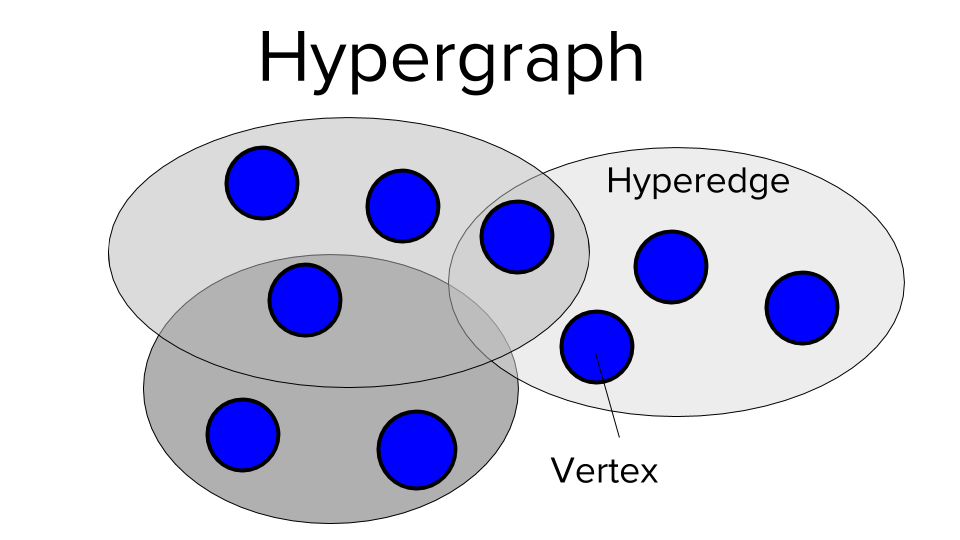[2-min CS Concepts] What is a Hypergraph?You most likely know graphs. The web graph consists of websites connected via hyperlinks. The websites are graph vertices and the hyperlinks are graph edges. Each graph edge connects exactly two vertices.

What happens if you drop this limitation and allow each edge to connect an arbitrary number of vertices? Simple: you get a hypergraph.

In the hypergraph, each hyperedge connects an arbitrary number of hypervertices instead of only two. So the hypergraph model allows you to model group relations instead of only binary relations.

For example, WhatsApp is a group-based communication technology. The group sizes race from two to thousands of members. Thus, hypergraphs are an excellent modeling abstraction for the WhatsApp social network.

Deepen your conceptual code understanding with the Finxter web app.